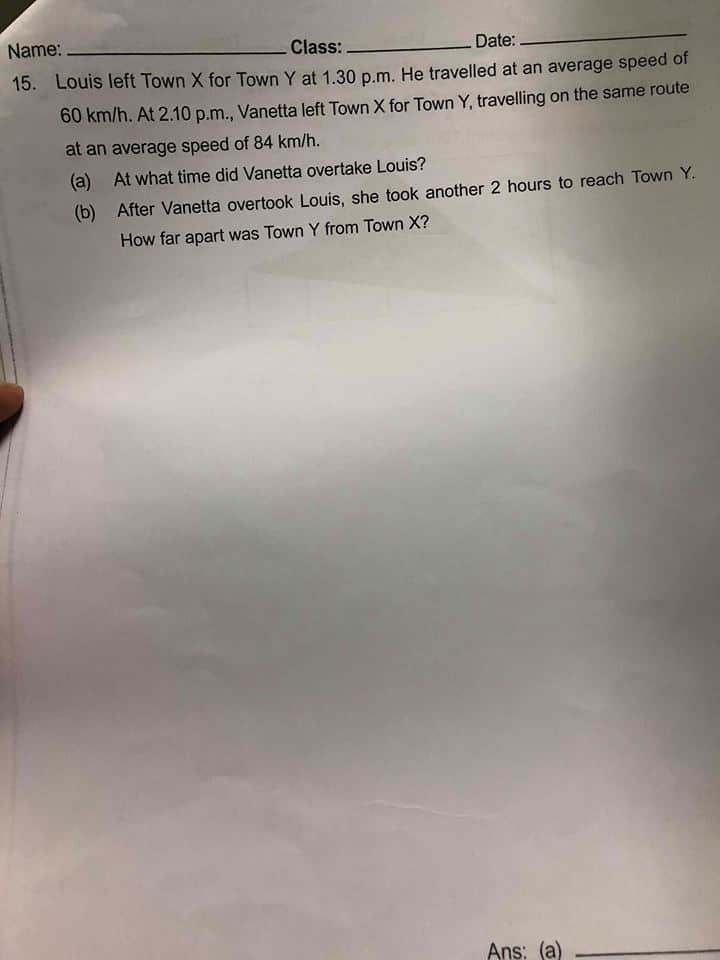# Questionhelp pls

This is a very interesting and useful set of topics, thank you for sharing! subway surfers

0 Replies 0 Likes

The distance for the headstart = 40/60 X 60 = 40 km.

Venatta is faster by 24 km/h, which is the relative speed she will use to close the gap of 40km.
Time taken for Venetta to reach Loius = 40 /24 = 1 hr 40 mins.

Time of overtaking = 2.10pm + 1hr 40mins = 3:50 pm ###

b) From the overtaking point she took another 2 hours to reach town Y and from town X to overtaking pt, she took 1 hr 40 min
Total time taken from X to Y = 2 + 1 hr 40 = 3 hours 40 mins = 11/3 hours

Distance = 84 X 11/3 = 308 km ##

The average speed is the total distance divided by the total travel time. Therefore, top ghostwriters, speed is distance divided by time. Instantaneous speed is the speed at which an object moves at any given moment.

0 Replies 0 Likes

This is a time speed distance question.

1. Louis start 40 minute = 2/3 hour earlier. Speed = 60

2. Vanette is catching up Louis. Speed = 84.

 Time Speed Distance Louis 1 2/3 60 40 Louis 2 1u 60 60u Vanette 1u 84 84u

40 + 60u = 84u

40 = 84u – 60u

24u = 40

1u = 40 ÷ 24 = 1 2/3 hour = 1 hr 40 min

a)

2 h 10 min + 1 hr 40 min = 3hr 50 min = 3.50 pm

b)

2 hr 10 min + 1 hr 40 mi  = 3 h 50 min = 3 5/6 hr

3 5/6 × 84 = 322 km.

😊

Note.

In time questions, use fraction instead of decimals.

2/3 hr = 2/3 × 60 = 40 minutes

50 min = 50/60 = 5/6 hours.

2 Replies 0 Likes

what is 1u in table?

40 + 60u = 84u?